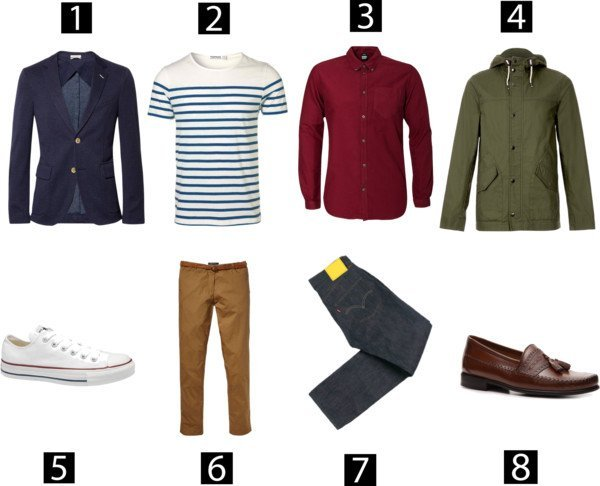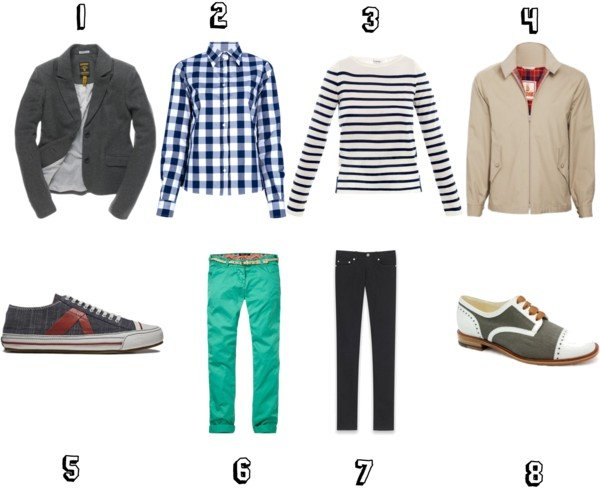# Mix & Match Mondays

A few weeks ago, a reader asked where to find affordable, versatile clothing that fits. In our answer, we featured the following classic pieces that can be mixed and matched to create a number of different ensembles. (Combine: 2+5+6; 2+5+7; 2+6+8; 2+7+8; 1+2+5+6; 1+2+5+7; 1+2+7+8; 2+3+5+6; 2+3+5+7; 2+3+7+8; 1+2+3+5+6; 1+2+3+5+7; 1+2+3+7+8; 3+5+6; 3+5+7; 3+6+8; 3+7+8; 1+3+5+6; 1+3+5+7; 1+3+6+8; 1+3+7+8; 1+2+3+5+6; 1+2+3+5+7; 1+2+3+6+8; 1+2+3+7+8; and just add 4 whenever you need!)We didn’t realize how popular this approach to dressing would be with our readers. So, we’ve decided to make Mix & Match Mondays a regular feature. Here’s this week’s dress by numbers: